CRR Tool
Capital Requirements Regulation (CRR)
Article 325bb

# Article 325bb - Expected shortfall risk measure

Status
In force
Selected consolidated version from
30/09/2021
Search all articles and keywords within this legal act

Article 325bb

Expected shortfall risk measure

1.

Institutions shall calculate the expected shortfall risk measure referred to in point (a) of Article 325ba(1) for any given date ‘t’ and for any given portfolio of trading book positions and non-trading book positions that are subject to foreign exchange or commodity risk as follows: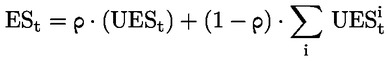where:

 ESt = the expected shortfall risk measure;
 i = the index that denotes the five broad categories of risk factors listed in the first column of Table 2 of Article 325bd;
 UESt = the unconstrained expected shortfall measure calculated as follows: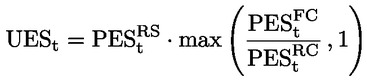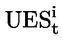=

the unconstrained expected shortfall measure for broad risk factor category i and calculated as follows: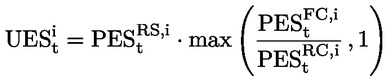ρ = the supervisory correlation factor across broad categories of risk; ρ = 50 %;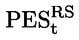= the partial expected shortfall measure that shall be calculated for all the positions in the portfolio in accordance with Article 325bc(2);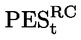= the partial expected shortfall measure that shall be calculated for all the positions in the portfolio in accordance with Article 325bc(3);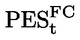= the partial expected shortfall measure that shall be calculated for all the positions in the portfolio in accordance with Article 325bc(4);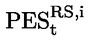= the partial expected shortfall measure for broad risk factor category i that shall be calculated for all the positions in the portfolio in accordance with Article 325bc(2);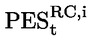= the partial expected shortfall measure for broad risk factor category i that shall be calculated for all the positions in the portfolio in accordance with Article 325bc(3); and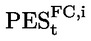= the partial expected shortfall measure for broad risk factor category i that shall be calculated for all the positions in the portfolio in accordance with of Article 325bc(4).
2.
Institutions shall only apply scenarios of future shocks to the specific set of modellable risk factors applicable to each partial expected shortfall measure, as set out in Article 325bc, when determining each partial expected shortfall measure for the calculation of the expected shortfall risk measure in accordance with paragraph 1.
3.
Where at least one transaction of the portfolio has at least one modellable risk factor which has been mapped to the broad risk factor category i in accordance with Article 325bd, institutions shall calculate the unconstrained expected shortfall measure for the broad risk factor category i and include it in the formula for the expected shortfall risk measure referred to in paragraph 1 of this Article.
4.
By way of derogation from paragraph 1, an institution may reduce the frequency of the calculation of the unconstrained expected shortfall measuresand of the partial expected shortfall measures,andfor all broad risk factor categories i from daily to weekly, provided that both of the following conditions are met:
(a)
the institution is able to demonstrate to its competent authority that calculating the unconstrained expected shortfall measuredoes not underestimate the market risk of the relevant trading book positions;
(b)
the institution is able to increase the frequency of calculation of,,andfrom weekly to daily where required by its competent authority.